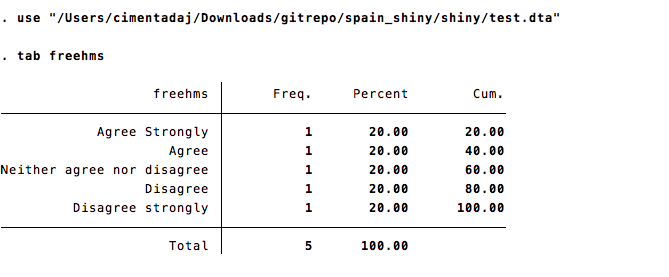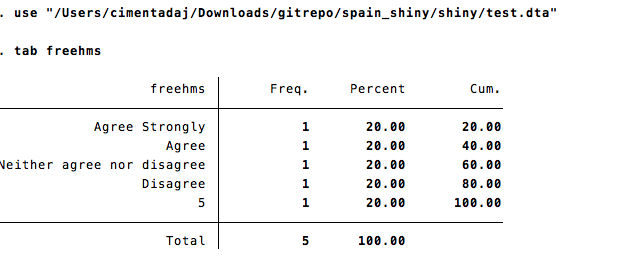# Saving missing categories from R to Stata

###### PUBLISHED ON MAR 16, 2019 — R

I’m finishing a project from the RECSM institute where we developed a Shiny application to download data from the European Social Survey with Spanish translated labels. This was one hell of a project since I had to build some wrappers around the Google Translate API to generate translations for over 1300 questions and stream line this to be interactive while users download the data. That’s a long story which I will not delve into.

This post is about a bug I found in the `haven` package while doing the project. The bug is simple to explain and I filed it in `haven` already:

Let’s define a labelled double with only one tagged NA value.

``````library(haven)
#> Warning: package 'haven' was built under R version 3.4.4

tst <-
labelled(
c(
1:5,
tagged_na("d")
),
c(
"Agree Strongly" = 1,
"Agree" = 2,
"Neither agree nor disagree" = 3,
"Disagree" = 4,
"Disagree strongly" = 5,
)
)

tst``````
``````## <Labelled double>
##      1     2     3     4     5 NA(d)
##
## Labels:
##  value                      label
##      1             Agree Strongly
##      2                      Agree
##      3 Neither agree nor disagree
##      4                   Disagree
##      5          Disagree strongly
``write_dta(data.frame(freehms = tst), "test.dta", version = 13)``

If I load this in Stata and type tab freehms, all labels are correct:Now, if I take the code above and add another tagged NA value, then `write_dta` drops the last label for some reason:

``````library(haven)

tst <-
labelled(c(1:5,
tagged_na('d'),
tagged_na('c')
),
c('Agree Strongly' = 1,
'Agree' = 2,
'Neither agree nor disagree' = 3,
'Disagree' = 4,
'Disagree strongly' = 5,
## And this
'Dont know' = tagged_na('c')
)
)

tst``````
``````## <Labelled double>
##      1     2     3     4     5 NA(d) NA(c)
##
## Labels:
##  value                      label
##      1             Agree Strongly
##      2                      Agree
##      3 Neither agree nor disagree
##      4                   Disagree
##      5          Disagree strongly
##  NA(c)                  Dont know``````
``write_dta(data.frame(freehms = tst), "test.dta", version = 13)``Well, the bug is evident (notice the 5 without a label?). However, since the project is on a deadline I had to come up with a solution. It’s very simple: avoid tagged NA’s but recode them as traditional labels. Here’s a solution:

``````library(sjlabelled)
library(sjmisc)

# Labels tags present in the ESS data
old_label_names <- c("a", "b", "c", "d")

# Grab the labels with tagged NA's with a regex
na_available <- unname(gsub("NA|\\(|\\)", "", get_na(tst, TRUE)))

# Identify which of the existent labels are actually valid ESS missings
which_ones_use <- old_label_names %in% na_available

# Subset only the ones which need recoding
value_code <- c(666, 777, 888, 999)[which_ones_use]
new_label_names <- c(".a", ".b", ".c", ".d")[which_ones_use]

# Recode them
for (i in seq_along(na_available)) {
tst <- replace_na(tst,
value = value_code[i],
na.label = new_label_names[i],
tagged.na = na_available[i]
)
}

tst``````
``````## <Labelled double>
##    1   2   3   4   5 888 999
##
## Labels:
##  value                      label
##      1             Agree Strongly
##      2                      Agree
##      3 Neither agree nor disagree
##      4                   Disagree
##      5          Disagree strongly
##    888                         .c
##    999                         .d``````

There we go. Those labels would clearly be interpreted as missings and Stata would read them as traditional labels (well, it’s not perfect, but it’s a workaround). What I did was wrap the above code into a function and apply it to all questions in all rounds (> 1300!).

``````recode_stata_labels <- function(x) {
# Labels tags present in the ESS data
old_label_names <- c("a", "b", "c", "d")

# Grab the labels with tagged NA's with a regex
na_available <- unname(gsub("NA|\\(|\\)", "", get_na(x, TRUE)))

# Identify which of the existent labels are actually valid ESS missings
which_ones_use <- old_label_names %in% na_available

# Subset only the ones which need recoding
value_code <- c(666, 777, 888, 999)[which_ones_use]
new_label_names <- c(".a", ".b", ".c", ".d")[which_ones_use]

for (i in seq_along(na_available)) {
x <- replace_na(x,
value = value_code[i],
na.label = new_label_names[i],
tagged.na = na_available[i]
)
}

x
}``````

Now, what happens if a `labelled` class only has tagged NA’s?

``````tst <-
labelled(c(1:5,
tagged_na('d'),
tagged_na('c')
),
c('No answer' = tagged_na('d'), 'Dont know' = tagged_na('c')))

tst``````
``````## <Labelled double>
##      1     2     3     4     5 NA(d) NA(c)
##
## Labels:
##  value     label
##  NA(c) Dont know``````
``recode_stata_labels(tst)``
``## Error: `x` must be a double vector``

That’s weird. I was in such a rush that I didn’t really want to debug the source code in `haven`. However, I had the intuition that this was related to the fact that there were only tagged NA’s as labels. How do I fixed it? Just add a toy label at the beginning of the function and remove it after the recoding.

``````recode_stata_labels <- function(x) {
# I add a random label (here) and delete it at the end (end of the function)
x <- add_labels(x, labels = c('test' = 111111))

# Note that this vector is in the same order as the `value_code` and `new_label_names`
# because they're values correspond to each other in this order.
old_label_names <- c("a", "b", "c", "d")

na_available <- unname(gsub("NA|\\(|\\)", "", sjlabelled::get_na(x, TRUE)))
which_ones_use <- old_label_names %in% na_available

value_code <- c(666, 777, 888, 999)[which_ones_use]
new_label_names <- c(".a", ".b", ".c", ".d")[which_ones_use]

for (i in seq_along(na_available)) {
x <- replace_na(x, value = value_code[i], na.label = new_label_names[i], tagged.na = na_available[i])
}

x <- remove_labels(x, labels = "test")

x
}

recode_stata_labels(tst)``````
``````## <Labelled double>
##    1   2   3   4   5 888 999
##
## Labels:
##  value label
##    888    .c
##    999    .d``````

There we are. The `replace_na` function is actually doing most of the work and I found it extremely useful (comes from the `sjmisc` package).